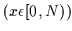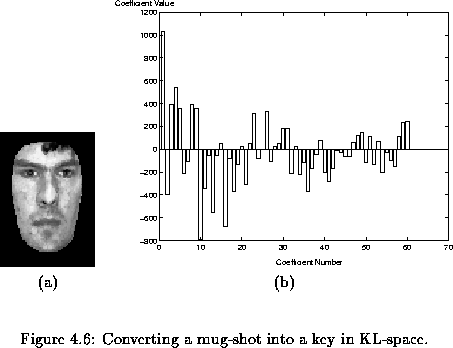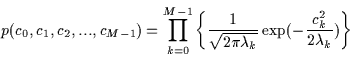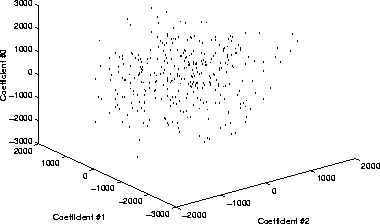Next: Decoding a Key into Up: Karhunen-Loeve Decomposition for Statistical Previous: Computing Eigenvalues and Eigenvectors

## Encoding Face Images with a Linear Combination Key

We now have a way of converting each vector in the dataset into a set of 60 scalars which will form a 60-dimensional key coding of the image. The key is composed of the scalar coefficients that determine the linear combination of eigenvectors to approximate the original image vector. The computation of the 60-coefficient key (c0, c1,..., c59) is performed using Equation. In Figure, we display the 60 scalar code representing one face from our training set. This encoding is performed for each face xin the database giving us a total of N 60-element vectors of the form (cx0, cx1, ..., cx59).With K-L decomposition, there is no correlation between the coefficients in the key (i.e., each dimension in the 60 dimensional space populated by face-points is fully uncorrelated). Consequently, the dataset appears as a multivariate random Gaussian distribution. The corresponding 60 dimensional probability density function is approximated in the L2 sense by Equation:(4.35)

The envelope of this Gaussian distribution is a hyperellipsoid  whose axis along each dimension is proportional to the eigenvalue of the dimension. In other words, the hyperellipsoid is thin'' in the higher-order dimensions and relatively wide in the lower-order ones. Although it is impossible to visualize the distribution in 60 dimensions, an idea of this arrangement can be seen in Figurewhich shows the distribution of the data set along the 3 first-order coefficients (associated with the 3 first-order eigenvectors).Next: Decoding a Key into Up: Karhunen-Loeve Decomposition for Statistical Previous: Computing Eigenvalues and Eigenvectors
Tony Jebara
2000-06-23